# Refraction through a rectangular glass slab - Physics - eSaral

Hey, do you want to learn about Refraction through a rectangular glass slab? If so. Then keep reading.

## Refraction through slab:

The Refractive index & thickness of the glass slab is µ & t respectively. One light ray AB incidents on a slab, Displacement produced, in emergent ray due to refraction.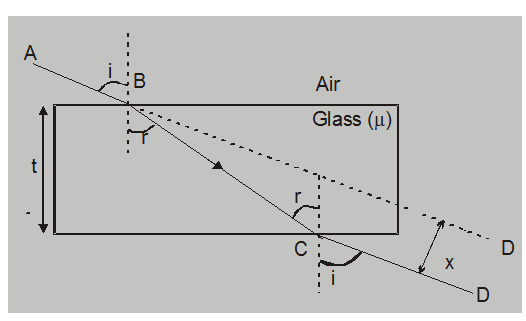$x=\frac{t \sin (i-r)}{\cos r}=t \sec r \sin (i-r)$

### (a) When an object is in denser medium & observer in rarer medium:

The thickness of a denser medium is t, in which an object is at a point O. Due to refraction, the image may be seen at a point I.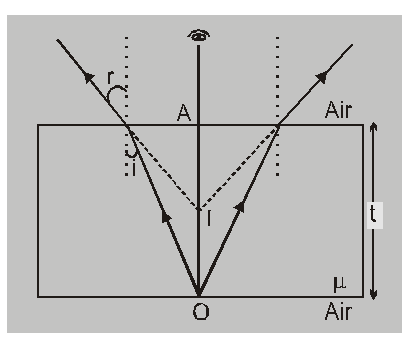Refractive index

$\mu=\frac{\text { Real depth }}{\text { Virtualdepth }}=\frac{\mathrm{AO}}{\mathrm{Al}}=\frac{\mathrm{t}}{\mathrm{Al}}$

Virtual depth $=\frac{t}{\mu}$

Virtual displacement $(\mathrm{OI})=\mathrm{OA}-\mathrm{Al}=\mathrm{t}\left(1-\frac{1}{\mathrm{\mu}}\right)$

### (b) Refraction through a successive slab of different thickness & refractive index.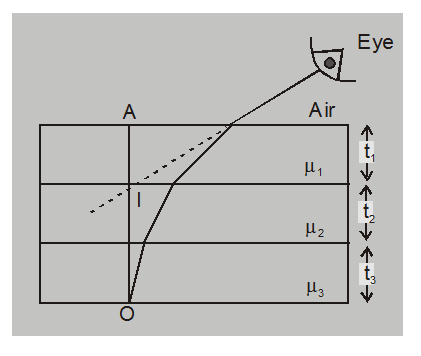Virtual depth $(\mathrm{Al})=\frac{\mathrm{t}_{1}}{\mu_{1}}+\frac{\mathrm{t}_{2}}{\mu_{2}}+\frac{\mathrm{t}_{3}}{\mu_{3}}+\ldots$

Virtual displacement (Ol) $=\mathrm{t}_{1}\left(1-\frac{1}{\mu_{1}}\right)+\mathrm{t}_{2}\left(1-\frac{1}{\mu_{2}}\right)+\mathrm{t}_{3}\left(1-\frac{1}{\mu_{3}}\right)+$............

### (c) When object & observer both are in rarer medium.

Let observer is in air & object is at a point O in air, as shown in the figure. A glass is there in between observer & object. Images forms at point I Refractive index of glass is $\mu$.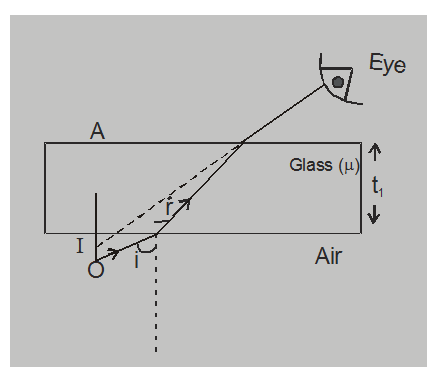Virtual displacement $=\mathrm{Ol}=\left(\mathrm{t}-\frac{1}{\mathrm{\mu}}\right)$

### (d) When an object in a rarer medium & Observer in the denser medium.

The Refractive index of water is $\mu .$ Observer is in water, Image may be seen at a point I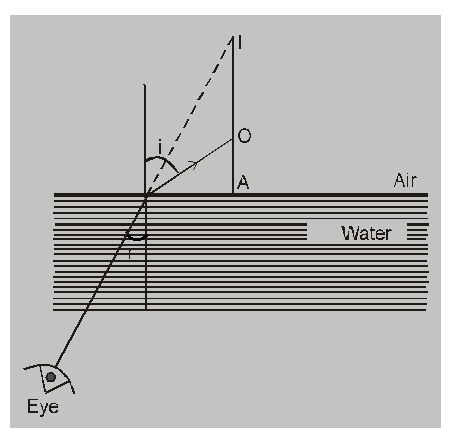when an object at a point O is viewed.

$\frac{\text { Re al height }}{\text { Virtual height }}=\frac{1}{\mu}$

Virtual displacement $(\mathrm{O} \mid)=\mathrm{Al}-\mathrm{AO}=(\mu-1) \mathrm{AO}$

So, that's all from this article. I hope you get the idea about Refraction through a rectangular glass slab. If you found this article informative then please share it with your friends. If you have any confusion related to this topic then feel free to ask in the comments section down below.

For a better understanding of this chapter, please check the detailed notes of Ray Optics. To watch Free Learning Videos on physics by Saransh Gupta sir Install the eSaral App.